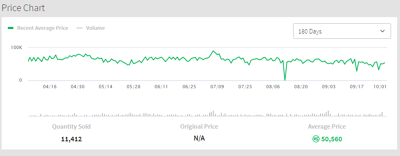24,122 Pages

The Recent Average Price, often abbreviated as RAP is what is calculated in trades and an average selling price of each individual limited item.

## Overview

A limited item's RAP is reflected by how much it sells for on the avatar shop, trades for, and its daily amount of copies sold averagely. A limited can sell for above or below its RAP, both affecting its 'Robux' value. If it sells above its corresponding RAP, then it will increase, if it sells below, then it will decrease; depending how much it is sold for.A RAP graph as displayed on a limited item page.

### Simple Formula

In order to find how much an item will raise or lower can easily be interpreted. Firstly, subtract the lowest selling price from the current RAP, and divide that result by 10, then round it to a whole number. When calculated, the number that you receive is estimated to be how much Robux will effectively ascend or descend the limited's RAP when it is sold.

#### Ascending Example

A limited unique hat's RAP is 12,500 Robux

```15,000 - 12,500 = 2,500

2,500 ÷ 10 = 250

12,500 + 250 = 12,750
```

#### Descending Example

A limited face's RAP is 35,000 Robux and its lowest selling price is 30,000 Robux. Once it has been sold, the formula is applied as follows:

```35,000 - 30,000 = 5,000

5,000 ÷ 10 = 500

35,000 - 500 = 34,500
```
Community content is available under CC-BY-SA unless otherwise noted.